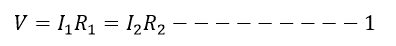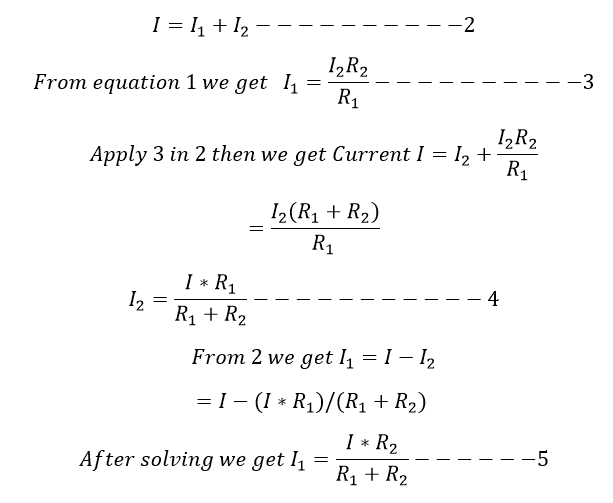# Current divider rule:

The current divider is used to find out the flowing current through the parallel circuit. Such a parallel circuit can be called as current divider since the current is divided into two branches of the circuit.

Let consider the branch currents are I1 and I2.

In the similar way of the voltage divider, we can calculate the expression for branch current.

The voltage drop across in the parallel branches is equal to the source voltage V. Hence,Apply KCL, hence the total current